Regular engagement with ICSE Class 9 Maths Solutions S Chand Chapter 12 Area Theorems Ex 12 can boost students confidence in the subject.

## S Chand Class 9 ICSE Maths Solutions Chapter 12 Area Theorems Ex 12

Question 1.
In the figure, ABC is a triangle in which D is the mid-point of BC and E is the mid-point of AD.
Prove that the area of ∆BED = $$\frac { 1 }{ 4 }$$ area of ∆ABC.Solution:
Given : In ∆ABC, D is the mid-point of BC and E is the mid point of AD
To prove : area of ABED = $$\frac { 1 }{ 4 }$$ area (∆ABC)
Construction : Join BE
Proof: In ∆ABC,
are ∆AABD = $$\frac { 1 }{ 2 }$$ area ∆ABC … (i)
In ∆ADB, BE is the median
area (∆BED) = $$\frac { 1 }{ 2 }$$ area (∆ABD)
= $$\frac { 1 }{ 2 }$$ x $$\frac { 1 }{ 2 }$$ area (∆ABC)
= $$\frac { 1 }{ 4 }$$ area (∆ABC)
Hence proved.

Question 2.
Prove that a line joining the mid-points of the parallel sides of a trapezium divides it into two equal parts.
Solution:
Given : In trapezium ABCD, AB || DC
E and F are the mid-points of sides AB and DC respectively
E and F are joinedTo prove : EF divides the trapezium into two equal parts
Cosntruction : From F, draw FL ⊥ AB
Proof : Area of trapezium = $$\frac { 1 }{ 2 }$$ (sum of parallel sides x height)
= $$\frac { 1 }{ 2 }$$ (AB + DC) x FL … (i)
∵ EF divides the trapezium into two trapeziums
∴ area of trapezium (AEFD) = $$\frac { 1 }{ 2 }$$ (AE + DF) x FL
But AE = $$\frac { 1 }{ 2 }$$ AB and DF = $$\frac { 1 }{ 2 }$$ DC
∴ area = $$\frac { 1 }{ 2 }$$[$$\frac { 1 }{ 2 }$$AB + $$\frac { 1 }{ 2 }$$DC] x FL
= $$\frac { 1 }{ 2 }$$ x $$\frac { 1 }{ 2 }$$ (AB + DC) x FL
= $$\frac { 1 }{ 4 }$$(AB + DC) x FL … (ii)
From (i) and (ii).
area of trapezium AEFD = $$\frac { 1 }{ 2 }$$ area of trapezium ABCD
Hence EF divides the trapezium ABCD into two equal parts in area

Question 3.
Prove that the diagonals of any parallelogram divide it into four equal triangles.
Solution:
Given : ABCD is a parallelogram whose diagonals AC and BD bisect each other at O forming four triangles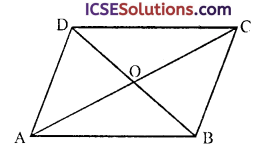To prove : Area of four triangles are equal
Proof: ∵ In a ||gm ABCD, diagonals bisect the ||gm into equal triangles
∴ AC bisects ||gm into two triangles equal in area
∴ area ∆ABC = area ∆ADC … (i)
In ∆ABC, 0 is the mid-point of AC
∴ BO is its median
∴ area ∆AOB = area ABOC … (ii)
Similarly, area ∆AOD = area ∆COD … (iii)
From (i), (ii) and (iii)
area ∆AOB = area ∆BOC = area ∆COD = area ∆DOA
Hence diagonals of a ||gm divides it into 4 equal mangles

Question 4.
The area of a triangle is equal to the area of a rectangle standing on the same base and between the same parallels lines.
Solution:
It is false.
∵ The area of a triangle is half to the area of the rectangle standing on the same base and between the same parallels lines.

Question 5.
In the figure, ABCD is a parallelogram. O is any point on the diagonal AC of the parallelogram. Show that the area of ∆AOB is equal to the area of ∆AOD.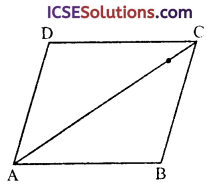Solution:
Given : In ||gm ABCD, AC is its diagonal and O is any point on AC
OB and OD are joined
To prove : area ∆AOB = area ∆AOD
Construction : Join BD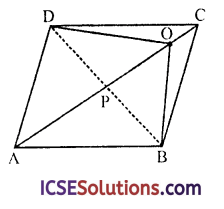Proof: ∵ Diagonals AC and BD bisect each other at P
∴ area ∆APB = area ∆APD … (i)
But in ∆OBD,
OP is the median (∵ P is mid point on BO)
∴ area ∆BOP = area ∆DOP … (ii)
area (∆APB + ∆PBO) = area (∆APD + ADOP)
⇒ area ∆AOB = area ∆AOD
Hence proved.

Question 6.
(a) Draw a rectangle ABCD with base 4 cm and diagonal BD = 5 cm. Measure CB. Take any point P on AD. Join BP. From C draw a line parallel to BP to meet AP produced at Q.
(b) What type of quadrilateral is PBCQ?
(c) Prove that the area of the rectangle ABCD is equal to that of the figure PBCQ.
(d) Prove that the area of the triangle PBC is half the area of the rectangle ABCD.
Solution:
Steps of constructions :
(a) (i) Draw a line segment AB = 4 cm.
(ii) Draw a perpendicular AX at A.
(iii) With centre B and radius 5 cnt, draw an arc which intersects AX at D.
(iv) With centres B and radius = AD and with centre D and radius = AB, draw arcs which intersect each other at C.
(v) Join DC, DB and BC.
ABCD is the required rectangle.
(vi) Take a point P on AD are join BP.
(vii) From C, draw a line parallel to BP which meets AX at Q.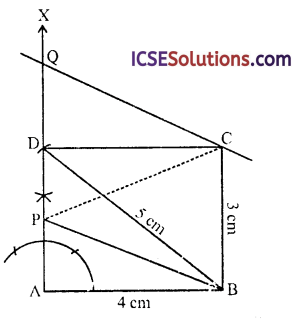(b) ∵ CQ || BP and AQ || BC
∴ PBCQ is a ||gm

(c) ∵ Rectangle ABCD and ||gm PBCQ lie on the same base BC and between the same parallels.
∴ area ABCD area PBCQ

(d) Join PC.
∵ APBC and rectangle ABCD lie on the same base BC and between the same parallels
area (APBC) = $$\frac { 1 }{ 2 }$$ area rectangle ABCD

Question 7.
In the figure, ABCD is a trapezium with AD and BC as parallel sides and ∠BAD = 90°. BD and AC cut at E. Prove that ∆ABE is equal in area to ACED.Solution:
Given : In trapezium ABCD, AD || BC and ∠BAD = 90°
Diagonals AC and BD intersect each other at E
To prove : area ∆ABE = area ∆CED
Proof: ∵ ∆ABD and ∆ACD lie on the same base AD and between the same parallel lines
∴ area ∆ABD = area ∆ACD
Subtracting area ∆AED from both sides
area ∆ABD – area ∆AED = area ∆ACD – area ∆AED
⇒ area ∆ABE = area ∆CED
Hence proved.

Question 8.
(i) Construct a parallelogram ABCD and parallelogram ABEF, where AB = 8 cm and the altitude of each parallelogram is 3 cm.
(ii) Prove that parallelogram ABCD has the same area as parallelogram ABEF.
(iii) Prove that triangle ABE has half the area of parallelogram ABCD.
Solution:
Steps of constructions :
(i) Draw a line segment AB = 8 cm.

(ii) At A, draw AL ⊥ AB and cut off AM = 3 cm.

(iii) Through M, draw XY ⊥ AB and produce it to both sides.
Now take two pairs of points CD = EF = 8 cm.

(iv) Join AD, BC and AF, BE.
Then ABCD and ABEF are required ||gms.

(v) ∵ ||gm ABCD and ||gm ABEF are on the same base AB and between the same parallel lines, then area ||gm ABCD = area ||gm ABEF.

(vi) JoinAE.
∵ ∆AEB and ||gm ABCD are on the same base and between the same parallel lines
∴ area ∆AEB = $$\frac { 1 }{ 2 }$$ area ||gm ABCD.
Hence proved.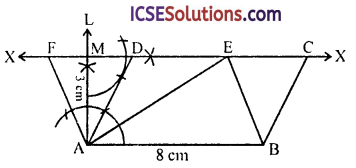Question 9.
In the figure, the area of the triangle ABC = 7.2 cm², CM = MB, and AL = 2 LB. Find the area of the triangle ALM.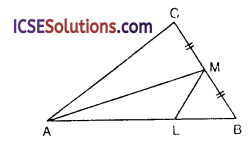Solution:
Given : In ∆ABC, M is a point on BC such that BM = MC. AM is joined L is a point on
AB such that AL = 2LB
LM is joined area of ∆ABC = 7.2 cm²
To find : Find the area of ∆ALM
AM is median of ∆ABC on BC
∴ area ABM = $$\frac { 1 }{ 2 }$$ area ∆ABC
∵ L is a point on AB such that AL = 2LB
∴ ∆AML and ∆LMB are along the same base and their vertice in same
∴ $$\frac{\text { area } \triangle \mathrm{ALM}}{\text { area } \triangle \mathrm{MLB}}=\frac{2}{1}$$
⇒ area ∆ALM = 2area ∆MLB
or area ∆ALM = $$\frac { 1 }{ 2 }$$ area ∆AMB
= $$\frac { 2 }{ 3 }$$($$\frac { 1 }{ 2 }$$area ∆ABC)
= $$\frac { 1 }{ 3 }$$ area ∆ABC
= $$\frac { 1 }{ 3 }$$ (7.2) cm² = 2.4 cm²

Question 10.
In figure, DE || BC. If DC and BE meet at O, prove that ABOD and ACOE are equal in area.Solution:
Given : In ∆ABC,
DE || BC
BE and CD meet at O
To prove : area (∆BOD) = area (∆COE)
Proof : ∆BCD and ∆BCE are on the same base BC and between the same parallel lines
∴ area (∆BCD) = area (∆COE)
Subtracting area (∆OBC) from both sides, we get
area ∆BCD – area ∆OBC = area ∆BCE – area ∆BOC
⇒ area (∆BOD) = area (∆COE)
Hence proved.

Question 11.
In the figure, squares are drawn on the side AB and the hypotenuse AC of the right triangle ABC. If BH is perpendicular to FG, prove that area of the square ABDE = area of the rectangle ARHF.Solution:
Given: ∆ABC is aright angled triangle, right angle at B. Square ABDE and ACGF are drawn on the side AB and hypotenuse AC of ∆ABC
BH ⊥ FG is drawn
BF and CE are joined
To prove: area sq. ABDE = area redt. ARHF
Proof: In ∆AEC and ∆ABF,
AE = AB (sides of square)
AC = AF (sides of square)
∠EAC = ∠BAF (each = 90° + ∠BAC)
∴ ∆AEC ≅ ∆ABF
∴ area ∆AEC = area ABAF … (i)
Now ∆AEC and square ABDE are on the same base AE and between the same parallels
∴ area ∆AEC = $$\frac { 1 }{ 2 }$$ area sq. ABDE … (ii)
Similarly ∆BAF and rect. ARHF are on the same base AF and between the same parallels
∴ area ABAF = $$\frac { 1 }{ 2 }$$ area rect. ARHF … (iii)
From (i), (ii) and (iii)
$$\frac { 1 }{ 2 }$$ area sq. ABDE = $$\frac { 1 }{ 2 }$$ area rect. ARHF
or area sq. ABDE = area rect. ARHF
Hence proved

Question 12.
The vertex A of a triangle ABC is joined to a point D on the side BC. The mid-point of AD is X. Prove that the area of triangle BXC is half the area of triangle ABC.
Solution:
Given : In ∆ABC,
D is any point on BC and X is midpoint of AD
BX and CX are joined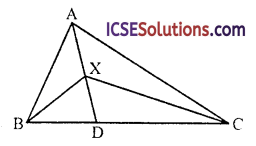To prove : area (∆BXC) = $$\frac { 1 }{ 2 }$$ area (∆ABC)
Proof: In ∆ABD, X is mid-point of AD
∴ BX is median
∴ area ABXD = $$\frac { 1 }{ 2 }$$ area ∆ABD … (i)
Similarly in ∆ACD,
CX is median
∴ area ∆DXC = $$\frac { 1 }{ 2 }$$ area ∆ADC … (ii)
area ∆BXD + area ∆DXC = $$\frac { 1 }{ 2 }$$ area ∆ABD + $$\frac { 1 }{ 2 }$$ area ∆ADC
⇒ area ∆BXC = $$\frac { 1 }{ 2 }$$ (area ∆ABD + area ∆ADC)
= $$\frac { 1 }{ 2 }$$ area ∆ABC
Hence area ∆BXC = $$\frac { 1 }{ 2 }$$ area ∆ABC
Hence proved.

Question 13.
In the figure, AR = RC and PC is parallel to BQ. Name a triangle equal to APCQ and a triangle equal in area to ∆APR, giving your reason. Hence prove that the areas of ∆APR, and quadrilateral PBCR are equal.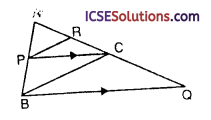Solution:
Given : In the figure, AR.= RC
PC || BQ
To find : Name a triangle equal to APCQ and a triangle equal in area to ∆APR
To prove : area of ∆APR = area of quadrilateral PBCR
Construction : Join PQProof:
(i) ∵ APCQ and APCB are on the same base PC and between the same parallel lines
∴ area ∆PCQ = area ∆PCB

(ii) In ∆APC, AR is median (∵ AR = RC)
area ∆APR = area ∆CPR

Question 14.
In the parallelogram ABCD, the side AB is produced to X, so that BX = AB. The line DX cuts BC at E. Prove that
(i) DBXC is a parallelogram;
(ii) area AED = twice area CEX.
Solution: Given : In ||gm ABCD, side AB is produced to X such that BX = AB
Line DX cuts BC at E
To prove :
(i) DBXC is a ||gm
(ii) area ABD = 2(area ACEX)
Construction : Join AE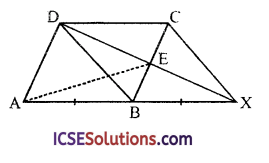Proof:
(i) ∵ ABCD is a ||gm
∴ AB ||DC or ABX || DC
∵ BX = AB = DC
∴ DBXC is a ||gm

(ii) In ||gm DBXC
∵ Diagonals DX and BC bisect each other at E
∴ area (∆CEX) = $$\frac { 1 }{ 4 }$$ area (||gm DBXC) … (i)
∵ ||gm ABCD and ||gm DBCX are on the same base DC and between the same parallels area ||gm
∴ ABCD = area ||gm DBXC
∴ area (∆CEX) = $$\frac { 1 }{ 4 }$$ area ||gm ABCD
⇒ area ||gm ABCD = 4 area (∆CEX)
∵ ∆AED and ||gm ABCD are on the same base AD and between the same parallels
∴ area ∆AED = $$\frac { 1 }{ 2 }$$ area ||gm ABCD
= $$\frac { 1 }{ 2 }$$ (4 area ACEX)
= 2 [area (∆CEX)]
Hence area ∆AED = 2 area ∆CEX

Question 15.
A point T is taken on the side PQ of the parallelogram PQRS, and the line ST and RQ are produced to meet at V. Prove that the triangles VSQ and VTR are equal in area.
Solution:
Given : In ||gm PQRS
T is any point on PQ
ST and RQ are produced to meet at V
SQ and TR are joined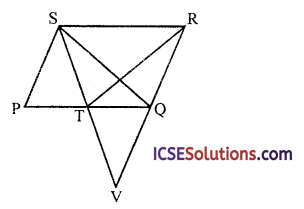To prove : area ∆VSQ = area ∆VTR
Proof : ∆STR and ∆SQR are on the same base SR and between the same parallels
∴ area ∆SQR = area ∆STR
area ∆SQR + area ∆TVQ = area ∆STR + area ∆TVQ
⇒ area ∆SVR = area ∆TVR
or area ∆VSQ = area ∆VTR
Hence proved.

Question 16.
ABCD is a parallelogram and E is any point on AB. If DE produced meets CB produced at F, prove that
(i) the area of triangle ADF = the area of the triangle DEC;
(ii) the area of triangle AEF = the area of triangle BEC.
Solution:
Given : In ||gm ABCD, E is any point on AB DE and CB are produced to meet at F
AF and CE are joined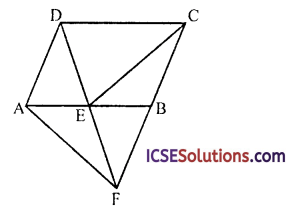To prove :
(ii) area ∆AEF = area ∆BEC
Proof:
(i) ∆ADF and ||gm ABCD are on the same base AD and between the same parallels
∴ area ∆ADF = $$\frac { 1 }{ 2 }$$ area ||gm ABCD … (i)
Similarly ADEC and ||gm ABCD are on the same base DC and between the same parallels
∴ area ADEC = $$\frac { 1 }{ 2 }$$ area ||gm ABCD … (ii)
From (i) and (ii),

(ii) ∵ area ∆ADF = $$\frac { 1 }{ 2 }$$ area ||gm ABCD
⇒ area ∆AED + area ∆AEF = $$\frac { 1 }{ 2 }$$ area ||gm ABCD … (iii)
and area ∆DEC = $$\frac { 1 }{ 2 }$$ area ||gm ABCD
⇒ area ∆AED + area ∆BEC = $$\frac { 1 }{ 2 }$$ area ||gm … (iv)
From (iii) and (iv),
area ∆AED + area ∆AEF = area ∆AED + area ABEC
⇒ area ∆AEF = area ∆BEC
Hence proved.

Question 17.
A trapezium ABCD has sides AB and DC parallel. A straight line parallel to the diagonal AC cuts AB at E and BC at F. Prove that triangles AED and ACF are equal in area.
Solution:
In trapezium ABCD, AB || DC, EF || diagonal AC is drawn which meets AB in E and BC in F
Join AE, AF
To prove : area ∆AED = area ∆ACF
Construction : Join EC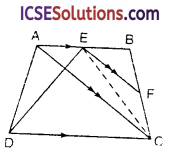Proof : ∆ADE and ∆AEC are on the same base AE and between the same parallel lines
∴ area ∆ADE = area ∆AEC … (i)
Similarly ∆AEC and ∆ACF are on the same base AC and between the same parallel lines area
∴ ∆AEC = area ∆ACF … (ii)
From (i) and (ii),
or area ∆AED = area ∆ACF
Hence proved.

Question 18.
Prove that the parallelogram formed by joining the mid-points of the adjacent sides of a quadrilateral is half of the latter.Solution:
EFGH is a ||gm formed by joining the mid-points of sides AB, BC, CD and DA of a quad. ABCD
To prove: area (||gmEFGH) = $$\frac { 1 }{ 2 }$$ area ABCD
Construction : Join AC and AG
Proof: In ∆ACD, G is the mid-point of CD
∴ AG is its median
∴ area (∆AGD) = $$\frac { 1 }{ 2 }$$ area (∆ACD) … (i)
Similarly GH is the median of ∆AGD
∴ area (∆AGH) = $$\frac { 1 }{ 2 }$$ area (∆AGD) … (ii)
From (i) and (ii),
area(∆AGH) = $$\frac { 1 }{ 2 }$$ area(∆AGD)
= $$\frac { 1 }{ 2 }$$ x $$\frac { 1 }{ 2 }$$ area (∆ACD)
= $$\frac { 1 }{ 4 }$$ area (∆ACD) … (iii)
But ∆AGH and ||gm PQGH are on the same base GH and between the same parallel lines
∴ area (∆ABH) = $$\frac { 1 }{ 2 }$$ area (||gm PQGH) … (iv)
From (iii) and (iv),
$$\frac { 1 }{ 4 }$$ area (∆ACD) = $$\frac { 1 }{ 2 }$$ area (||gm PQGH)
⇒ area (∆ACD) = 2 area (||gm PQGH)
Similarly we can prove that
area (∆BCD) = 2 area (||gm PQFE)
2 area (||gm PQGF) x 2 area (||gm PQFE)
= area (∆ACD) + area (ABCD)
⇒ 2 [area (||gm PQBH) + area (||gm PQFE)] = area ABCD
⇒ 2 area (||gm EFGH) = area ABCD
⇒ area (||gm EFGH) = $$\frac { 1 }{ 2 }$$ area ABCD
Hence proved.

Question 19.
In the figure, AB || DC || EF, AD || BC and ED || FA. Prove that the area of DEFH is equal to the area of ABCD.Solution:
Given : In the figure, AB || DC || EF
AD || BC and ED || FA
To prove : area (DEFH)
= area (ABCD)
Proof : ||gm ABCD and ||gm ADEG are on the same base AD and between the same parallel lines
∴ area (ABCD) = area (ADEG) … (i)
Similarly ||gm ADEG and ||gm DEFH are on the same base DE and between the same parallel lines
∴ area (||gm ADEG) = area (||gm DEFH) … (ii)
From (i) and (ii),
area (DEFH) = area (ABCD)
Hence proved.

Question 20.
In the figure, M and N are the mid-points of the sides DC and AB of the parallelogram ABCD and the area of the parallelogram ABCD is 36 cm².
(i) State the area of the triangle BEC.
(ii) Name the parallelogram which is equal in area to the triangle BEC.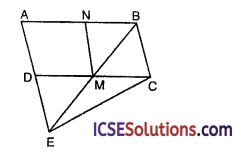Solution:
Given : ABCD is a ||gm
M and N are mid-points of sides AB and DC
respectively
MN is joined
BM and AD are produced to meet at E EC is joined
Area of ||gm ABCD = 36 cm²
(i) State the area of ∆BEC
(ii) Name the parallelogram which is equal in area to the ∆BEC
Proof:
(i) ∆BEC and ||gm ABCD are on the same base BC and between the same parallel lines AE || BC
∴ area (ABEC) = $$\frac { 1 }{ 2 }$$ area (||gm ABCD)
= $$\frac { 1 }{ 2 }$$ x 36 = 18 cm²

(ii) ∵ M and N are the mid-points of AB and DC
∴ ||gm ADMN = ||gm MCBN = $$\frac { 1 }{ 2 }$$ ||gm ABCD
= $$\frac { 1 }{ 2 }$$ x 36 cm² = 18 cm²
∴ area (ABEC) = area ||gm ADMN = area ||gm MCBN